## CLTV using RFM and Probability Models

By: Ram on Oct 23, 2020

"Customer Lifetime Value is the discounted cumulative cash flows that a customer brings to the firm over the entire duration of the relationship with the company" (Kumar 2006)

"Customer Lifetime Value is a monetary value that represents the amount of revenue or profit a customer will give the company over the period of the relationship" (Source).

## Why do we need CLV?

• Helps align acquisition costs with long-term value generation instead of revenue and/or volume
• Focus retention on the highest potential CLV increase
• Up/cross-sell based on potential incremental CLV
• Generate ROI via customer experience improvements
• Use CLV for the valuation of the firm
• Allows firms to assess the true worth of customers and to target their marketing efforts to the most profitable customers.

## Calculate Customer Lifetime Value (CLV)

There are several CLV calculation approaches available. The selection and relevance of the method or approach may depend on the context, complexity, and accuracy required.

1. Modeling CLV using RFM
2. Modeling CLV based on Probability Models
3. Modeling CLV using a combination of Econometric & ML models

In this tutorial, we will cover some of the simple scenarios using a sample dataset.

## Data and Context

In this blog, it is a retail and non-subscription based context for modeling CLV. We are using a dataset available on Kaggle. This is a dataset based on the transactions at the Brazilian e-commerce website - Olist Store.

The dataset has information about 100k orders from 2016 to 2018 made at multiple marketplaces in Brazil.

There are several different files and types of information available. We may use only customers, orders, and payment files.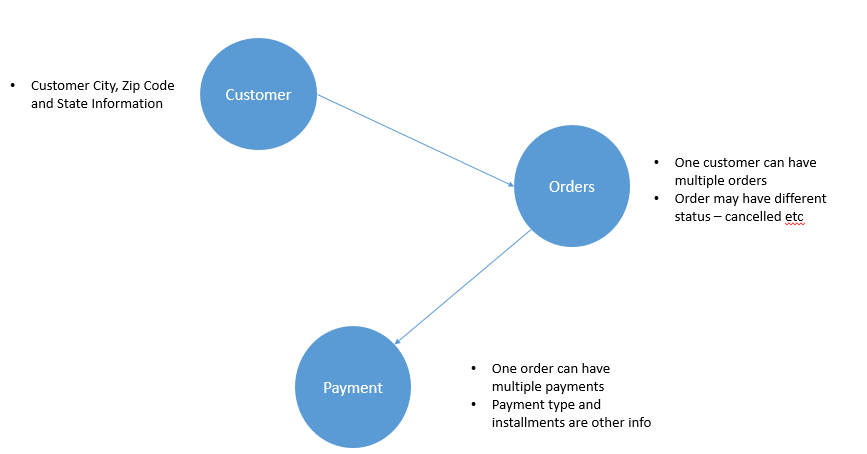`# Connect to Google drive `

`from google.colab import drive`

`drive.mount('/content/drive')`

`# Read files from Google Drive Folder`

`import pandas as pd`

`customers = pd.read_csv("/content/drive/My Drive/MICA/CLTV/olist_customers_dataset.csv.zip",sep=',', engine='python')`

`orders = pd.read_csv("/content/drive/My Drive/MICA/CLTV/olist_orders_dataset.csv.zip",sep=',', engine='python')`

`payments = pd.read_csv("/content/drive/My Drive/MICA/CLTV/olist_order_payments_dataset.csv.zip",sep=',', engine='python')`

### Customer Data

`customers.head()`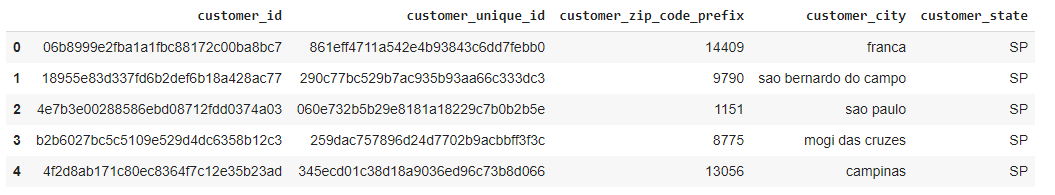Order Data

`orders.head()`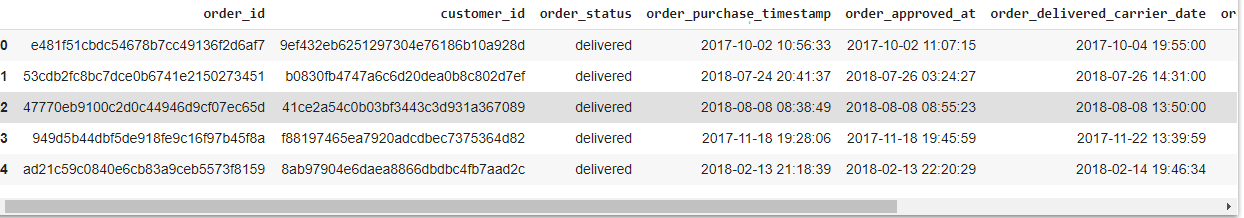We may want to see the distribution of the transactions across year and month to know a bit more about the data. Before that, we will just see the aggregated information.

`orders.info()`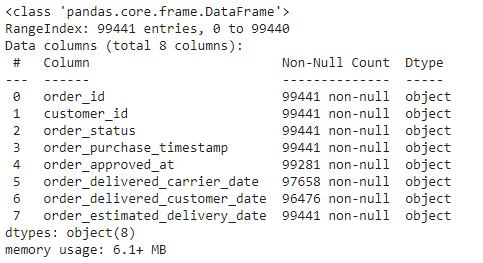`# Create order date`

`# 2018-08-02 12:06:07`

`orders['order_date'] = pd.to_datetime(orders.order_purchase_timestamp, format='%Y-%m-%d %H:%M:%S').dt.date`

`orders.head()`

`import seaborn as sns`

`import matplotlib.pyplot as plt`

`orders['order_mon_year'] = orders['order_date'].apply(lambda x: x.strftime('%Y-%m'))`

`order_mon_year=orders['order_mon_year'].sort_values()`

`print(order_mon_year.value_counts())`

`orders.sort_index().reset_index()`

`plt.figure(figsize=(8,4))`

`ax = sns.countplot(order_mon_year)`

`ax.set_xticklabels(ax.get_xticklabels(), `

`                   rotation=90,`

`                   ha="right")`

`plt.tight_layout()`

`plt.show()`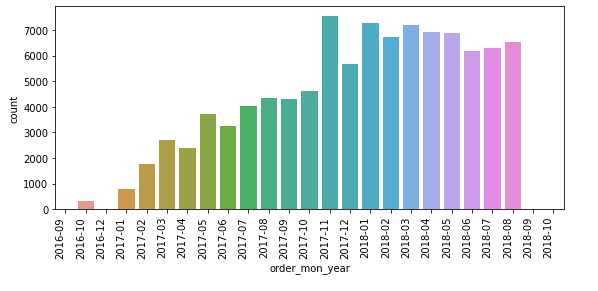### Payment Data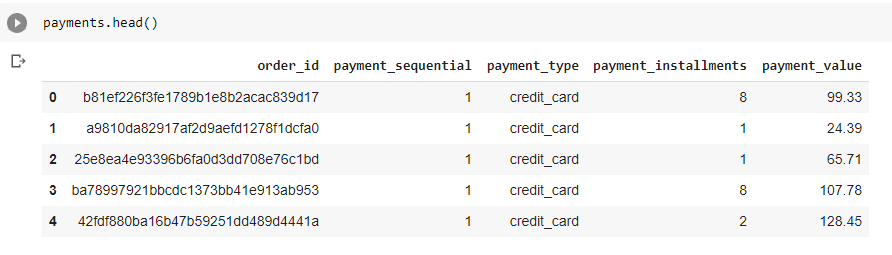### Analysis  Sample

Though the transactions are across 3 years - 2016, 2017, and 2018, we will consider Jan-2017 to Aug-2018 data for the analysis.

`# Select orders between two dates`

`flag_analysis = (orders['order_date'] >= pd.to_datetime('2017-01-01',format="%Y-%m-%d")) & (orders['order_date'] <= pd.to_datetime('2018-08-31',format="%Y-%m-%d"))`

`orders = orders.loc[flag_analysis]`

`orders.head()`

### Distribution of order status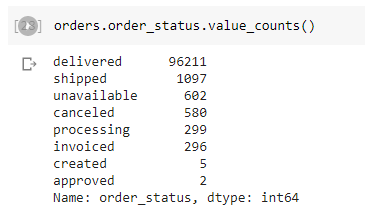We may want to keep only delivered orders for the analysis.

`orders=orders[orders['order_status']=='delivered']`

## RFM Analysis

R - Recency: How recently did a customer buy? If a customer has not bought for a long, it is very less likely the customer will buy

F - Frequency: How frequently does a customer buys from a shop? If a customer buys frequently, the customer is more likely to buy it again.

M - Monetary: What is the total value of purchases? If a customer buys of more value, higher chances of the customer coming back again. Some customers buy a week's need at once.

So for the RFM analysis, based on the current data, we need to calculate the following:

• Days between the recent day (30-Apr-2018) and the last purchase day for a customer
• Number of orders for each customer
• Purchase value or payment value for each customer

First, we need to join customers, orders, and payment data.

`# Customer Orders - Purchase `

`import pandas as pd`

`cust_orders = pd.merge( customers, orders, on='customer_id',how='inner')`

`cust_orders_purchase = pd.merge(cust_orders, payments, on='order_id', how='inner')`

`cust_orders_purchase.head()`

Now, we need to aggregate the data and calculate Recency (days between the latest purchase and the analysis end date), frequency (count of orders), and total value of purchases.

`import numpy as np`

`cust_rfm=cust_orders_purchase.groupby('customer_unique_id').agg({'order_date': lambda date: (order_date.max() - order_date.min()).days,`

`                                                                      'order_date': [np.max],`

`                                        'order_id': lambda num: len(num),`

`                                        'payment_value': lambda val: val.sum()})`

`cust_rfm.head()`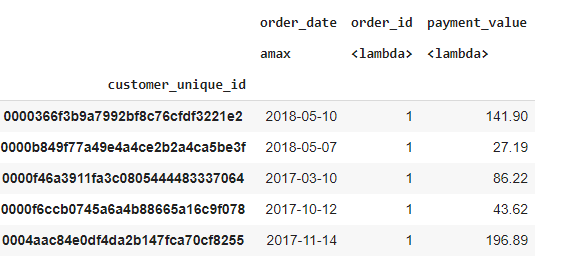`cust_rfm=cust_rfm.reset_index()`

`cust_rfm.columns = ['customer_id','latest_order_date','frequency','monetary']`

`cust_rfm.head()`

Recency - we need to calculate days between the latest purchase date and the analysis date (31-Oct-2018)

`import numpy as np`

`cust_rfm['recency'] =  ((pd.to_datetime('2018-08-31', format="%Y-%m-%d")-pd.to_datetime(cust_rfm['latest_order_date'])) /np.timedelta64(1, 'D'))`

### Summary Statistics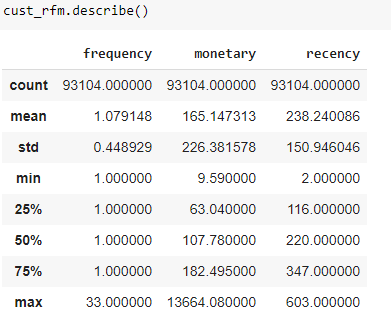### Distribution of Recency, Frequency, and Monetary Values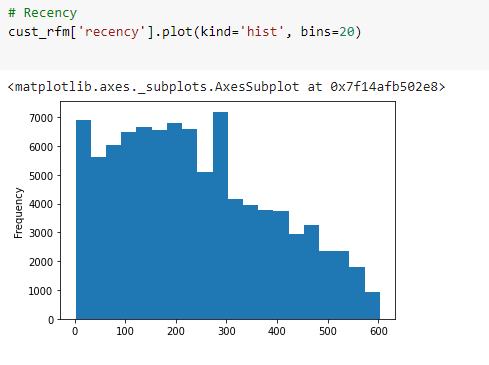### Frequency

`# Frequency`

`cust_rfm['frequency'].plot(kind='hist', bins=50)`

`cust_rfm['frequency'].value_counts()`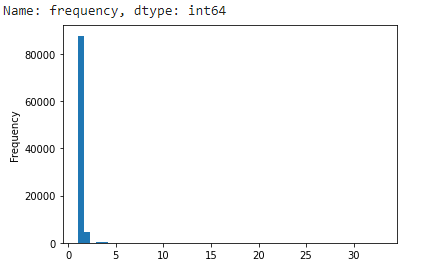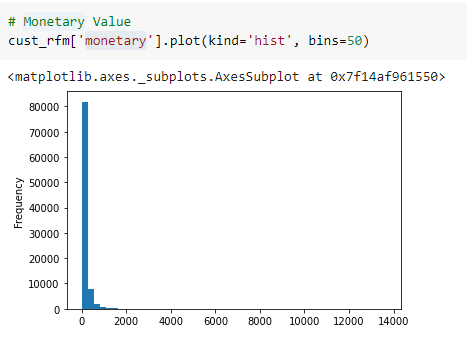### RFM for Customer Value

RFM based model is based on historical customer transactions. It is not a futuristic or predictive framework. But, it can definitely be a good lever to segment great customers from average or poor customers.

We can aggregate ranking based on RFM Measures and aggregate these to find a customer value lever. This may not be a great absolute measure but can play useful relative measures of customer value.

Recency - low value, the customer has recently made a purchase. So, the low value should have a better score

Frequency - Higher is better

Monetary - Higher is better

`# Recency Rank `

`cust_rfm['recency_score'] = cust_rfm['recency'].rank(method='dense', ascending=False).astype(int)`

`cust_rfm['frequency_score'] = cust_rfm['frequency'].rank(method='dense', ascending=True).astype(int)`

`cust_rfm['monetary_score'] = cust_rfm['monetary'].rank(method='dense', ascending=True).astype(int)`

`cust_rfm.head()`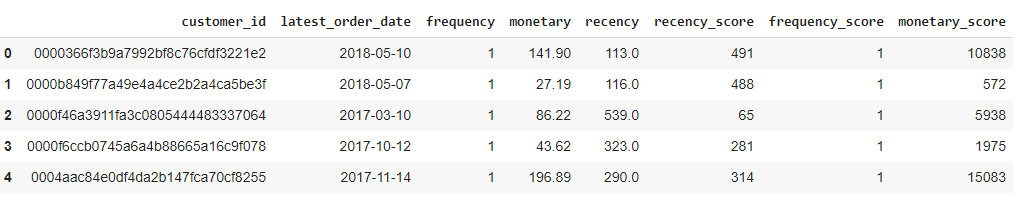The scale of values for all these 3 scores are different. So, we need to either standardize these scores or manage by weights.

`from sklearn.preprocessing import MinMaxScaler`

`cust_rfm_scaled = cust_rfm.loc[:,['recency_score',  'frequency_score','monetary_score']]`

`scaler = MinMaxScaler()`

`cust_rfm_scaled=scaler.fit_transform(cust_rfm_scaled)`

`cust_rfm_df = pd.DataFrame(cust_rfm_scaled)`

`cust_rfm_df.columns =['recency_scaled','frequency_scaled','monetary_scaled']`

`cust_rfm_df.head()`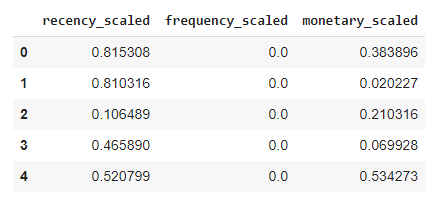### Weightage RFM for Customer Value

50% for Monetary and 25% for each Recency and Frequency

`cust_rfm_df['value_score'] = 0.25*cust_rfm_df['recency_scaled']+ 0.25*cust_rfm_df['frequency_scaled']+ 0.5*cust_rfm_df['monetary_scaled']`

We can create a few buckets based on the value score and we can see the purchase value and also see the spend in the future period.

Now, we can find the average profit per customer using % Margin and average spend across all customers.

## Probability Models for CLTV

The way to estimate CLV is

LTV = expected number of transaction * revenue per transaction * margin

In this approach, the number of transactions per period (for a customer), average revenue, or spend per transaction per period (for a customer), and margin % are required.

The first two comes from Probability Models and the margin % comes from business or finance.

the number of transactions per period is estimated based on Beta-Geometric/Negative Binomial Distribution Model (or BG/NBD)

The revenue per transaction is estimated based on the Gamma-Gamma model.

Use the same scenario which has been used for RFM

### Beta-Geometric/Negative Binomial Model

Estimating the number of transaction for a customer across periods (e.g. each month)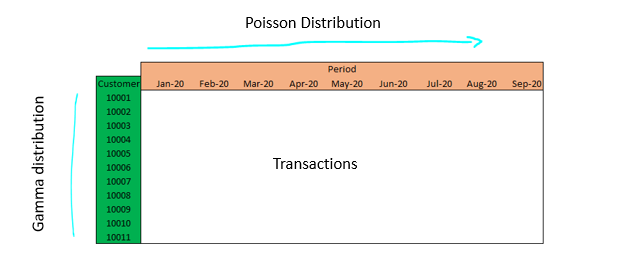• When a customer or user is active, the number of transactions in a time period of length t follows a Poisson distribution with transaction rate λ and using that transaction volume for the period t is estimated.
• The variation across customers (Y dimension) is assumed to follow Gamma distribution. The transaction rate across customers explains the difference in purchasing behavior. The Gamma distribution has parameters r (shape) and α (scale).
• Similar to transaction volume, inactive rate (the customer/user may become inactive after any transaction) distribution across the period is assumed to follow the Geometric distribution. Probability p and their dropout point (when they become inactive) is distributed between purchases with the Geometric distribution.
• The inactivity rate variation across users is assumed to follow the Beta distribution. The dropout probability has Beta distribution with the two shape parameters α and β.

Also, it is assumed that the transaction rate and dropout probability are independent of each other.

Source for these points and the Original Paper

`! pip install lifetimes`

`# Read Data`

`custs = pd.read_csv("/content/drive/My Drive/MICA/CLTV/olist_customers_dataset.csv.zip",sep=',', engine='python')`

`ords = pd.read_csv("/content/drive/My Drive/MICA/CLTV/olist_orders_dataset.csv.zip",sep=',', engine='python')`

`pays = pd.read_csv("/content/drive/My Drive/MICA/CLTV/olist_order_payments_dataset.csv.zip",sep=',', engine='python')`

`# Order Data`

`order_cols = ['customer_id', 'order_id', 'order_purchase_timestamp']`

`order = ords[order_cols]`

`order = order.set_index('customer_id')`

`order.drop_duplicates(inplace=True)`

`# Payment Data`

`pay_cols = ['order_id', 'payment_value']`

`payment = pays[pay_cols]`

`payment = payment.set_index('order_id')`

`payment.drop_duplicates(inplace=True)`

`# Customer Data`

`cust_cols = ['customer_id', 'customer_unique_id']`

`customer = custs[cust_cols]`

`customer = customer.set_index('customer_id')`

### Prepare Transaction Data

`# Orders and Customers`

`transactions = pd.concat([order,customer], axis=1, join='inner')`

`transactions.reset_index(inplace=True)`

`# Keep only relevant columns`

`cols = ['customer_unique_id', 'order_id','order_purchase_timestamp']`

`transactions = transactions[cols]`

`# Change to datetime`

`transactions['order_purchase_timestamp'] = pd.to_datetime(transactions['order_purchase_timestamp'])`

`transactions['order_date'] = transactions.order_purchase_timestamp.dt.date`

`transactions['order_date'] = pd.to_datetime(transactions['order_date'])`

`# Drop and keep relevant`

`cols = ['customer_unique_id','order_id', 'order_date']`

`transactions = transactions[cols]`

`# Rename`

`transactions.columns = ['CUSTOMER_ID','order_id', 'ORDER_DATE']`

`transactions.info()`

`display(transactions.sample(5))`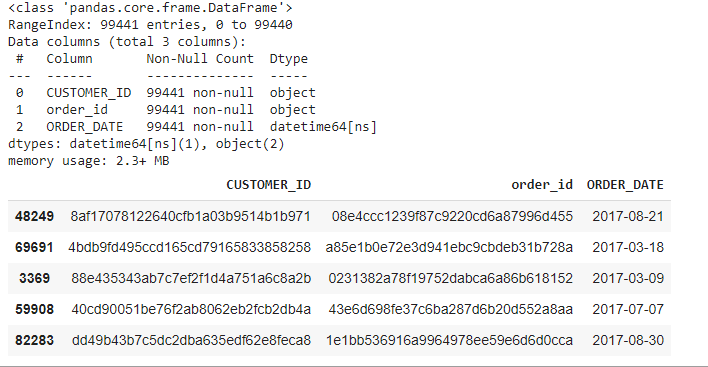`# Payment Transactions`

`pay_transactions = pd.merge (order, payment, on='order_id', how='left')`

`pay_transactions = pd.merge(pay_transactions,transactions, on = 'order_id', how='inner')`

`pay_transactions.head()`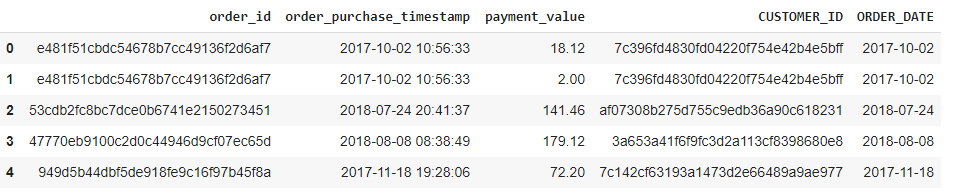`transactions_val=pay_transactions.groupby('CUSTOMER_ID').agg({'payment_value': lambda t: t.sum()`

`                                       })`

`transactions = pd.merge (transactions,transactions_val, on ='CUSTOMER_ID', how='inner')`

### RFM Matrix based on transactions

`from lifetimes.utils import calibration_and_holdout_data`

`summary_cal_holdout = calibration_and_holdout_data(transactions, `

`                                                   customer_id_col = 'CUSTOMER_ID', `

`                                                   datetime_col = 'ORDER_DATE', `

`                                                   freq = 'D', #days`

`                                        calibration_period_end='2018-06-30',`

`                                        observation_period_end='2018-09-28' )`

`summary_cal_holdout.head()`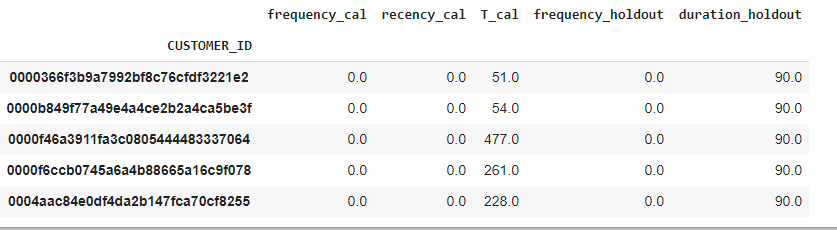### Recency and T

We need to keep track of two intervals - Days between Purchases (if there are repeat purchases) and second, the days between the last purchase and end of the analysis period.

Recency: Days between purchases. This is equal to the duration between a customer’s first purchase and their latest purchase.

Days Since First Purchase (T) - Find the days between the end of the analysis date and the first purchase day for each customer. This is one day a measure of customer period with the firm.

### Calculate Monetary Values

`summary_cal_holdout = pd.merge (summary_cal_holdout,transactions_val, on ='CUSTOMER_ID', how='inner')`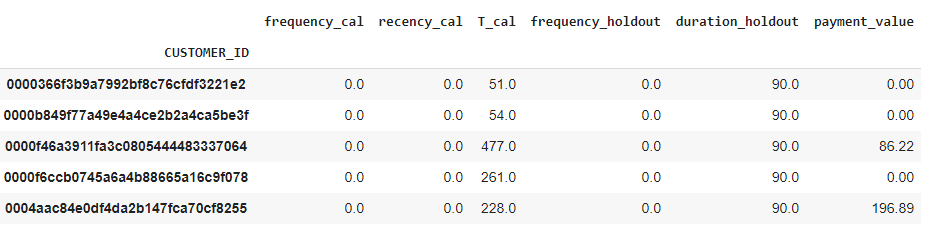### Visualizing our frequency/recency matrix

`from lifetimes.plotting import *`

`from lifetimes.utils import *`

`from lifetimes import BetaGeoFitter`

`from scipy.stats import gamma, beta`

`bgf = BetaGeoFitter(penalizer_coef=0.0)`

`bgf.fit(summary_cal_holdout['frequency_cal'], summary_cal_holdout['recency_cal'], summary_cal_holdout['T_cal'], )`

`print (bgf)`

`from lifetimes.plotting import plot_frequency_recency_matrix`

`import matplotlib.pyplot as plt`

`fig = plt.figure(figsize=(10,6))`

`plot_frequency_recency_matrix(bgf)`

`plt.show()`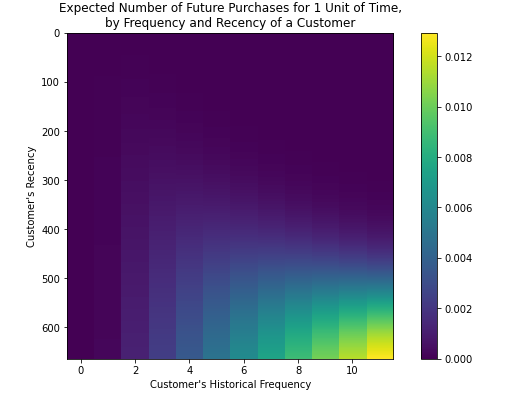### Fit the Model for Transaction Volume

`from lifetimes import ModifiedBetaGeoFitter`

`mbgnbd = ModifiedBetaGeoFitter(penalizer_coef=0.01)`

`mbgnbd.fit(summary_cal_holdout['frequency_cal'], `

`        summary_cal_holdout['recency_cal'], `

`        summary_cal_holdout['T_cal'],`

`       verbose=True)`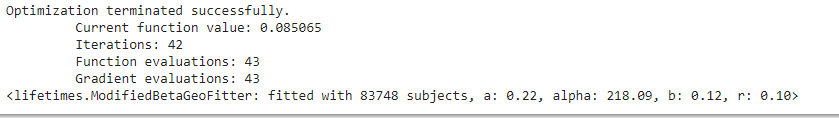### Transaction Value Estimation using the Gamma-Gamma model

`returning_customers_summary = summary_cal_holdout[(summary_cal_holdout['frequency_cal'] > 0) & (summary_cal_holdout['payment_value'] > 0)]`

`returning_customers_summary[['payment_value', 'frequency_cal']].corr()`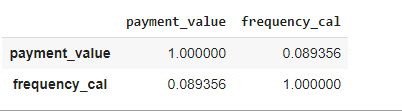`returning_customers_summary['payment_value'].hist()`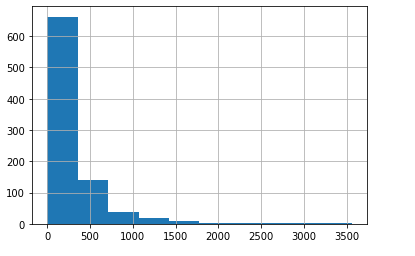### Checking Missing Values

`returning_customers_summary['payment_value'].isnull().sum()`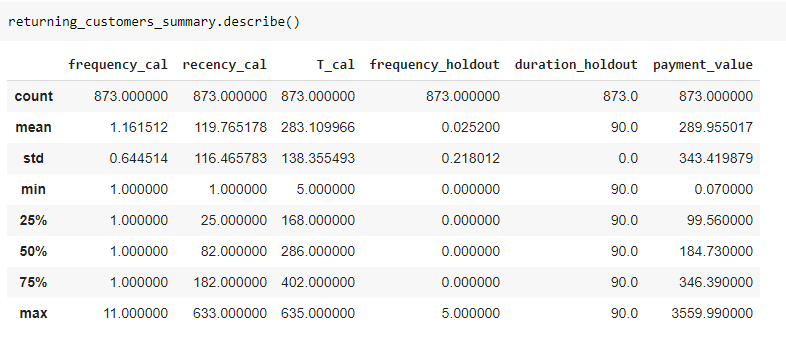gg = GammaGammaFitter(penalizer_coef = 0.01)

gg.fit(returning_customers_summary['frequency_cal'],

returning_customers_summary['payment_value'],

verbose=True)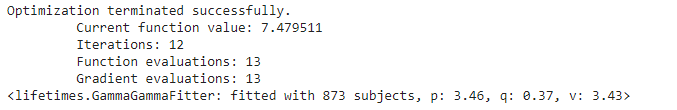`t = 90 # days to predict in the future `

`summary_cal_holdout['predicted_purchases'] = mbgnbd.conditional_expected_number_of_purchases_up_to_time(t, `

`                                                                                      summary_cal_holdout['frequency_cal'], `

`                                                                                      summary_cal_holdout['recency_cal'], `

`                                                                                      summary_cal_holdout['T_cal'])`

`summary_cal_holdout['p_alive'] = mbgnbd.conditional_probability_alive(summary_cal_holdout['frequency_cal'], `

`                                                                         summary_cal_holdout['recency_cal'], `

`                                                                         summary_cal_holdout['T_cal'])`

`summary_cal_holdout['p_alive'] = np.round(summary_cal_holdout['p_alive'] / summary_cal_holdout['p_alive'].max(), 2)`

### Estimating Customer Life Time Value

We can estimate customer lifetime value using a Discounted Rate. We can give this as input.

`summary_cal_holdout['clv'] = gg.customer_lifetime_value(`

`   mbgnbd, #the model to use to predict the number of future transactions`

`   summary_cal_holdout['frequency_cal'],`

`   summary_cal_holdout['recency_cal'],`

`   summary_cal_holdout['T_cal'],`

`   summary_cal_holdout['payment_value'],`

`   time=3, # months`

`   discount_rate=0 #0.0025 # = 0.03/12 monthly discount rate ~ 3% annually`

`)`

`summary_cal_holdout['clv'] += (-1*summary_cal_holdout['clv'].min())`

`display(summary_cal_holdout.sample(2).T)`

Display sample CLTV at a customer level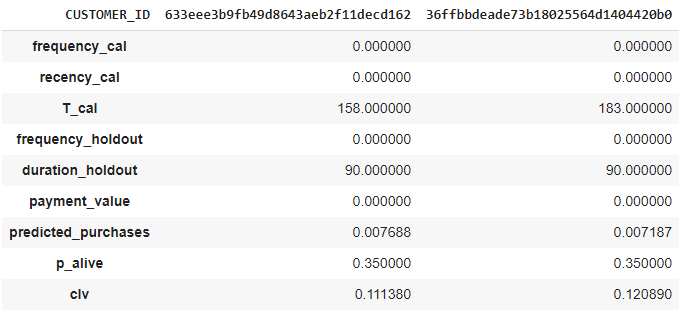### Model evaluation

The performance of the CLTV model can be evaluated by comparing actual and predicted values.

`from lifetimes.plotting import plot_period_transactions`

`ax = plot_period_transactions(mbgnbd, max_frequency=7)`

`ax.set_yscale('log')`

`sns.despine();`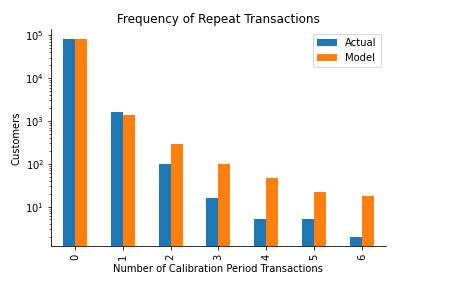`from lifetimes.plotting import plot_calibration_purchases_vs_holdout_purchases`

`plot_calibration_purchases_vs_holdout_purchases(mbgnbd, summary_cal_holdout)`

`sns.despine();`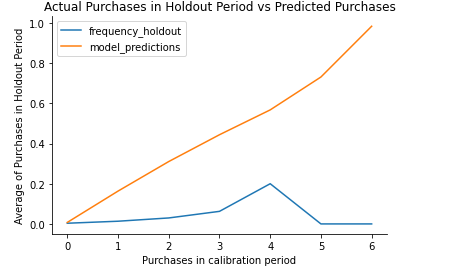### Predicted Transactions with Time

Get expected and actual repeated cumulative transactions.

`from lifetimes.utils import expected_cumulative_transactions`

`t = (transactions.ORDER_DATE.max() - transactions.ORDER_DATE.min()).days`

`df = expected_cumulative_transactions(mbgnbd, transactions, 'ORDER_DATE', 'CUSTOMER_ID', t)`

`from datetime import datetime`

`cal = datetime.strptime('2018-06-30', '%Y-%m-%d')`

`from lifetimes.plotting import plot_cumulative_transactions`

`t = (transactions.ORDER_DATE.max() - transactions.ORDER_DATE.min()).days`

`t_cal = (cal - transactions.ORDER_DATE.min()).days`

`plot_cumulative_transactions(mbgnbd, transactions, 'ORDER_DATE', 'CUSTOMER_ID', t, t_cal, freq='D')`

`sns.despine()`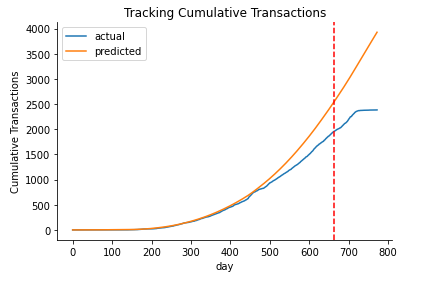` import numpy as np`

` print("Expected conditional average revenue: %s, Average revenue: %s" % (`

` np.round(gg.conditional_expected_average_profit(`

`     returning_customers_summary['frequency_cal'],`

`     returning_customers_summary['payment_value']).mean(), 2),`

`     np.round(returning_customers_summary[returning_customers_summary['frequency_cal']>0]['payment_value'].mean(), 2)`

`))`

Tags
Most PopularJun 18, 2020Jul 23, 2020Jun 19, 2020Jun 19, 2021